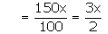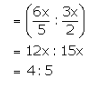# Two numbers are respectively 20% and 50% more than a third number

Two numbers are respectively 20% and 50% more than a third number. The ratio of the two numbers is:

Let the third number be x.

Then, the first number is 120% of xThe second number is 150% of xTherefore, Ratio of first two numbers: